## Basic Principles of Modeling Physical Networks

### Overview of the Physical Network Approach to Modeling Physical Systems

Simscape™ software is a set of block libraries and special simulation features for modeling physical systems in the Simulink® environment. It employs the Physical Network approach, which differs from the standard Simulink modeling approach and is particularly suited to simulating systems that consist of real physical components.

Simulink blocks represent basic mathematical operations. When you connect Simulink blocks together, the resulting diagram is equivalent to the mathematical model, or representation, of the system under design. Simscape technology lets you create a network representation of the system under design, based on the Physical Network approach. According to this approach, each system is represented as consisting of functional elements that interact with each other by exchanging energy through their ports.

These connection ports are nondirectional. They mimic physical connections between elements. Connecting Simscape blocks together is analogous to connecting real components, such as pumps, valves, and so on. In other words, Simscape diagrams mimic the physical system layout. If physical components can be connected, their models can be connected, too. You do not have to specify flow directions and information flow when connecting Simscape blocks, just as you do not have to specify this information when you connect real physical components. The Physical Network approach, with its Through and Across variables and nondirectional physical connections, automatically resolves all the traditional issues with variables, directionality, and so on.

The number of connection ports for each element is determined by the number of energy flows it exchanges with other elements in the system, and depends on the level of idealization. For example, a fixed-displacement hydraulic pump in its simplest form can be represented as a two-port element, with one energy flow associated with the inlet (suction) and the other with the outlet. In this representation, the angular velocity of the driving shaft is assumed constant, making it possible to neglect the energy exchange between the pump and the shaft. To account for a variable driving torque, you need a third port associated with the driving shaft.

An energy flow is characterized by its variables. Each energy flow is associated with two variables, one Through and one Across (see Variable Types for more information). Usually, these are the variables whose product is the energy flow in watts. They are called the basic, or conjugate, variables. For example, the basic variables for mechanical translational systems are force and velocity, for mechanical rotational systems—torque and angular velocity, for hydraulic systems—flow rate and pressure, for electrical systems—current and voltage.

The following example illustrates a Physical Network representation of a double-acting hydraulic cylinder.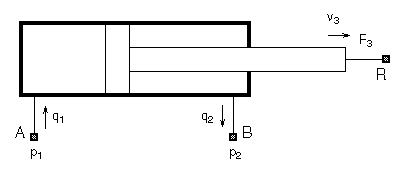The element is represented with three energy flows: two flows of hydraulic energy through the inlet and outlet of the cylinder and a flow of mechanical energy associated with the rod motion. It therefore has the following three connector ports:

• A — Hydraulic conserving port associated with pressure `p1` (an Across variable) and flow rate `q1` (a Through variable)

• B — Hydraulic conserving port associated with pressure `p2` (an Across variable) and flow rate `q2` (a Through variable)

• R — Mechanical translational conserving port associated with rod velocity `v3` (an Across variable) and force `F3` (a Through variable)

See Connector Ports and Connection Lines for more information on connector port types.

### Variable Types

Physical Network approach supports two types of variables:

• Through — Variables that are measured with a gauge connected in series to an element.

• Across — Variables that are measured with a gauge connected in parallel to an element.

The following table lists the Through and Across variables associated with each type of physical domain in Simscape software:

Physical DomainAcross VariableThrough Variable
ElectricalVoltageCurrent
GasAbsolute pressure and temperatureMass flow rate and energy flow rate
HydraulicGauge pressureVolumetric flow rate
Isothermal liquidAbsolute pressureMass flow rate
MagneticMagnetomotive force (mmf)Flux
Mechanical rotationalAngular velocityTorque
Mechanical translationalTranslational velocityForce
Moist AirAbsolute pressure, temperature, specific humidity (water vapor mass fraction), and trace gas mass fractionMixture mass flow rate, mixture energy flow rate, water vapor mass flow rate, and trace gas mass flow rate
ThermalTemperatureHeat flow
Thermal liquidAbsolute pressure and temperatureMass flow rate and energy flow rate
Two-phase fluidAbsolute pressure and specific internal energyMass flow rate and energy flow rate

### Building the Mathematical Model

Through and Across variables associated with all the energy flows form the basis of the mathematical model of the block.For example, the model of a double-acting hydraulic cylinder shown in the previous illustration can be described with a simple set of equations:

`${F}_{3}={p}_{1}·{A}_{1}-{p}_{2}·{A}_{2}$`

`${q}_{1}={A}_{1}·{v}_{3}$`

`${q}_{2}={A}_{2}·{v}_{3}$`

where

 q1,q2 Flow rates through ports A and B, respectively (Through variables) p1,p2 Gauge pressures at ports A and B, respectively (Across variables) A1,A2 Piston effective areas F3 Rod force (Through variable) v3 Rod velocity (Across variable)

The model could be considerably more complex, for example, it could account for friction, fluid compressibility, inertia of the moving parts, and so on. For all these different mathematical models, however, the element configuration (that is, the number and type of ports and the associated Through and Across variables) would remain the same, meaning that the Physical Network approach lets you substitute models of different levels of complexity without introducing any changes to the schematic. For example, you can start developing your system by using the Resistive Tube block from the Foundation library, which accounts only for friction losses. At a later stage in development, you may want to account for fluid compressibility. You can then replace it with a Hydraulic Pipeline block, available with Simscape Fluids™ block libraries, or, depending on your application, even with a Segmented Pipeline block if you also need to account for fluid inertia. This modeling principle is called incremental modeling.

### Direction of Variables

Each variable is characterized by its magnitude and sign. The sign is the result of measurement orientation. The same variable can be positive or negative, depending on the polarity of a measurement gauge.

Elements with only two ports are characterized with one pair of variables, a Through variable and an Across variable. Since these variables are closely related, their orientation is defined with one direction. For example, if an element is oriented from port A to port B, it implies that the Through variable (TV) is positive if it “flows” from A to B, and the Across variable is determined as AV = AVA – AVB, where AVA and AVB are the element node potentials or, in other words, the values of this Across variable at ports A and B, respectively.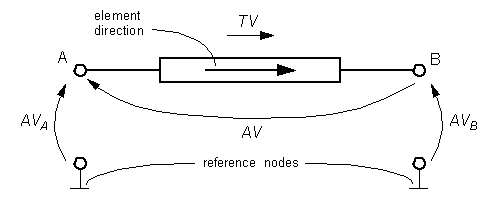This approach to the direction of variables has the following benefits:

• Provides a simple and consistent way to determine whether an element is active or passive. Energy is one of the most important characteristics to be determined during simulation. If the variables direction, or sign, is determined as described above, their product (that is, the energy) is positive if the element consumes energy, and is negative if it provides energy to a system. This rule is followed throughout the Simscape software.

• Simplifies the model description. Symbol `A → B` is enough to specify variable polarity for both the Across and the Through variables.

• Lets you apply the oriented graph theory to network analysis and design.

As an example of variables direction rules, let us consider the Ideal Force Source block. In this block, as in many other mechanical blocks, port C is associated with the source reference point (case), and port R is associated with the rod.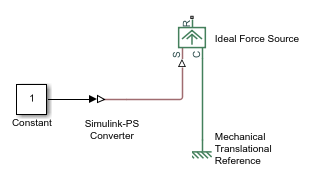The block positive direction is from port C to port R. This means that the force is positive if it acts in the direction from C to R, and causes bodies connected to port R to accelerate in the positive direction. The relative velocity is determined as `v` = `vC``vR`, where `vR`, `vC` are the absolute velocities at ports R and C, respectively, and it is negative if velocity at port R is greater than that at port C. The power generated by the source is computed as the product of force and velocity, and is negative if the source provides energy to the system.

Definition of positive direction is different for different blocks. Check the block source or the block reference page if in doubt about the block orientation and direction of variables.

All the elements in a network are divided into active and passive elements, depending on whether they deliver energy to the system or dissipate (or store) it. Active elements (force and velocity sources, flow rate and pressure sources, etc.) must be oriented strictly in accordance with the line of action or function that they are expected to perform in the system, while passive elements (dampers, resistors, springs, pipelines, etc.) can be oriented either way.

### Connector Ports and Connection Lines

Simscape blocks may have the following types of ports:

• Physical conserving ports — Nondirectional ports (for example, hydraulic or mechanical) that represent physical connections and relate physical variables based on the Physical Network approach.

• Physical signal ports — Unidirectional ports transferring signals that use an internal Simscape engine for computations.

Each of these ports and connections between them are described in greater detail below.

#### Physical Conserving Ports

Simscape blocks have special conserving ports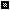. You connect conserving ports with physical connection lines, distinct from normal Simulink lines. Physical connection lines have no inherent directionality and represent the exchange of energy flows, according to the Physical Network approach.

• You can connect conserving ports only to other conserving ports of the same type.

• The physical connection lines that connect conserving ports together are nondirectional lines that carry physical variables (Across and Through variables, as described above) rather than signals. You cannot connect physical lines to Simulink ports or to physical signal ports.

• Two directly connected conserving ports must have the same values for all their Across variables (such as pressure or angular velocity).

• You can branch physical connection lines. When you do so, components directly connected with one another continue to share the same Across variables. Any Through variable (such as flow rate or torque) transferred along the physical connection line is divided among the multiple components connected by the branches. How the Through variable is divided is determined by the system dynamics.

For each Through variable, the sum of all its values flowing into a branch point equals the sum of all its values flowing out.

Each type of physical conserving ports used in Simscape blocks uniquely represents a physical modeling domain. For a list of port types, along with the Through and Across variables associated with each type, see the table in Variable Types.

For improved readability of block diagrams, each Simscape domain uses a distinct default color and line style for the connection lines. For more information, see Domain-Specific Line Styles.

#### Physical Signal Ports

Physical signal ports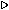carry signals between Simscape blocks. You connect them with regular connection lines, similar to Simulink signal connections. Physical signal ports are used in Simscape block diagrams instead of Simulink input and output ports to increase computation speed and avoid issues with algebraic loops. Physical signals can have units associated with them. You specify the units along with the parameter values in the block dialogs and Property Inspector, and Simscape software performs the necessary unit conversion operations when solving a physical network.

Simscape Foundation library contains, among other sublibraries, a Physical Signals block library. These blocks perform math operations and other functions on physical signals, and allow you to graphically implement equations inside the physical network.

Physical signal lines also have a distinct style and color in block diagrams, similar to physical connection lines. For more information, see Domain-Specific Line Styles.